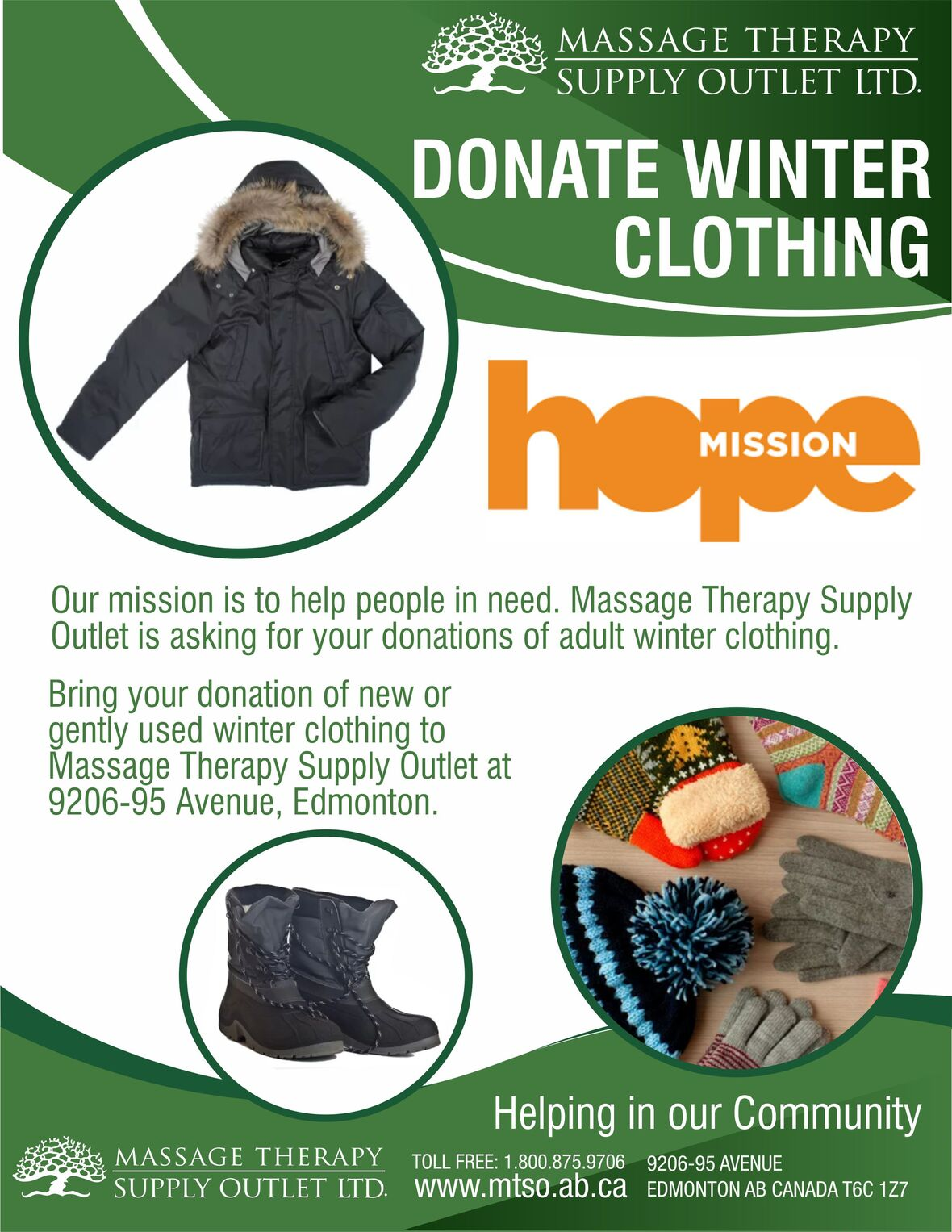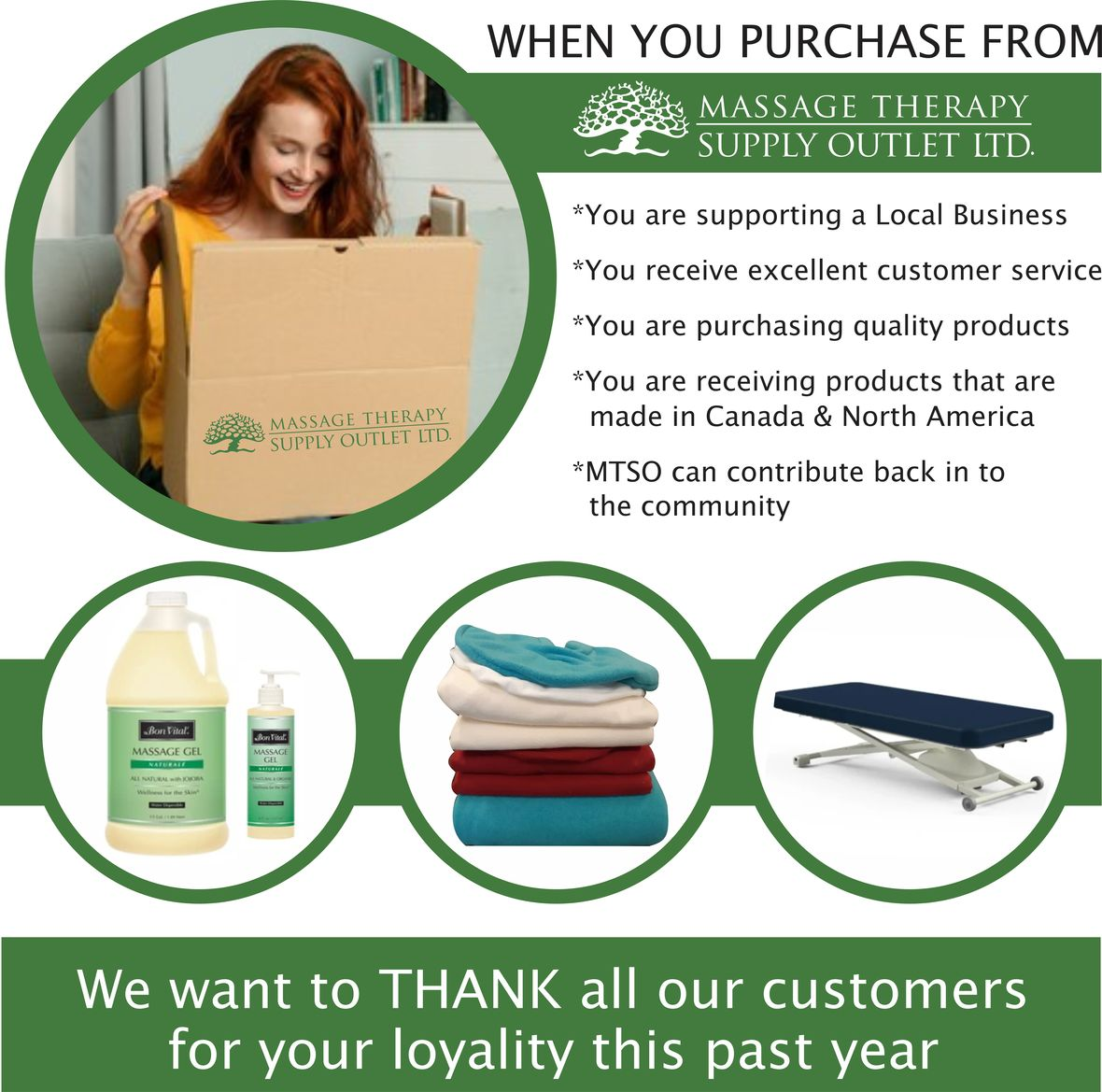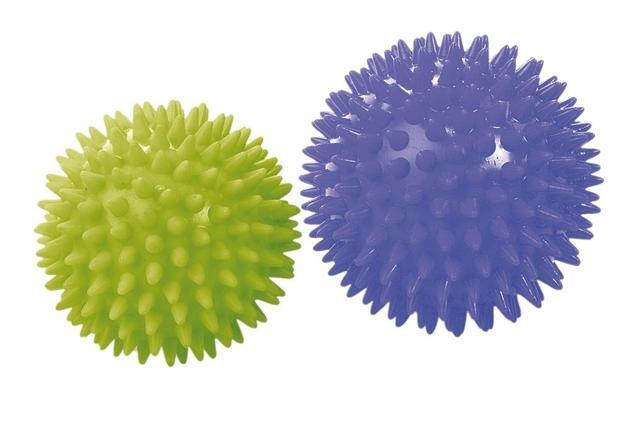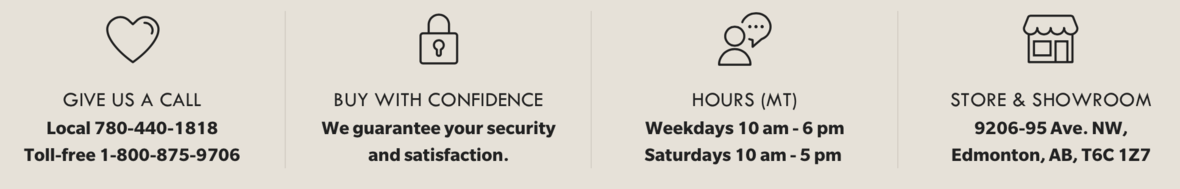## MTSO December NewsNow that the excitements of the Festive Season gather speed & sparkle around us, remember self-care. Maybe seek out your favourite massage therapist for a session.. or exchange treatments with gift cards for the new year!

Here are some simple Essential Oil Holiday Blends to diffuse to freshen the air & add cheer.

As the weather chills during this season of giving, we are still gathering winter clothing donations for the Hope Mission. Please bring an article of new or gently used adult clothing for someone in need.

As this crazy year draws to a close, please accept our sincere thanks for your loyal ongoing support of our small local business.

From all of us at MTSO Ltd., we wish you a wonderful Holiday Season & a Happy 2022 of renewed prosperity!”

 table div table+table+table+table div table{width:100%;padding:0}table div table+table+table+table div table img{width:96.23%;padding:0;float:none}table div table+table+table+table div table td{width:100%;padding:0 1.88% 18px}/* styles */table div table+table+table+table+table+table div table{width:100%;padding:0}table div table+table+table+table+table+table div table img{width:96.23%;padding:0;float:none}table div table+table+table+table+table+table div table td{width:100%;padding:0 1.88% 18px}/* styles */table div table+table+table+table+table+table+table+table+table div table{width:100%;padding:0}table div table+table+table+table+table+table+table+table+table div table img{width:96.23%;padding:0;float:none}table div table+table+table+table+table+table+table+table+table div table td{width:100%;padding:0 1.88% 18px}/* styles */## Holiday Aromatherapy

 table div table+table+table+table+table+table+table+table+table+table+table div table{width:100%;padding:0}table div table+table+table+table+table+table+table+table+table+table+table div table table{padding:0;float:left!important;width:51.886%!important}table div table+table+table+table+table+table+table+table+table+table+table div table table+table td,table div table+table+table+table+table+table+table+table+table+table+table div table td{padding-left:0;padding-right:0}table div table+table+table+table+table+table+table+table+table+table+table div table table td{padding-left:0;padding-right:20px}table div table+table+table+table+table+table+table+table+table+table+table div table table+table{float:left!important;width:48.114%!important}/* styles */

## Our favourite seasonal blends

You'll love the beneficial effects of any of these delightful holiday blends - transform your space with nature's aromatic essences.

Link HERE to our essential oils for all the ingredients.

 table div table+table+table+table+table+table+table+table+table+table+table+table+table div table{width:100%;padding:0}table div table+table+table+table+table+table+table+table+table+table+table+table+table div table img{width:96.23%;padding:0;float:none}table div table+table+table+table+table+table+table+table+table+table+table+table+table div table td{width:100%;padding:0 1.88% 18px}/* styles *//* styles */ Click on each image to get more information and to purchase.
 table div table+table+table+table+table+table+table+table+table+table+table+table+table+table+table+table div table{width:100%;padding:0}table div table+table+table+table+table+table+table+table+table+table+table+table+table+table+table+table div table table{padding:0;float:left!important;width:45.283%!important}table div table+table+table+table+table+table+table+table+table+table+table+table+table+table+table+table div table table+table td,table div table+table+table+table+table+table+table+table+table+table+table+table+table+table+table+table div table td{padding-left:0;padding-right:0}table div table+table+table+table+table+table+table+table+table+table+table+table+table+table+table+table div table table td{padding-left:0;padding-right:20px}table div table+table+table+table+table+table+table+table+table+table+table+table+table+table+table+table div table table+table{float:left!important;width:54.717%!important}/* styles */Acu-Reflex Massage Balls \$5.95 ea
 table div table+table+table+table+table+table+table+table+table+table+table+table+table+table+table+table+table+table div table{width:100%;padding:0}table div table+table+table+table+table+table+table+table+table+table+table+table+table+table+table+table+table+table div table img{width:96.23%;padding:0;float:none}table div table+table+table+table+table+table+table+table+table+table+table+table+table+table+table+table+table+table div table td{width:100%;padding:0 1.88% 18px}/* styles */# POLAR FLEECE BLANKETS

\$35.00
Quit fighting your patients' shiver response! Throw another layer on with our custom made fleece blankets.

Locally produced massage linens for your practice: Cozy Fleece Blankets to keep your clients warm. Perfectly sized. 75" x 60"

 table div table+table+table+table+table+table+table+table+table+table+table+table+table+table+table+table+table+table+table+table+table div table{width:100%;padding:0}table div table+table+table+table+table+table+table+table+table+table+table+table+table+table+table+table+table+table+table+table+table div table img{width:96.23%;padding:0;float:none}table div table+table+table+table+table+table+table+table+table+table+table+table+table+table+table+table+table+table+table+table+table div table td{width:100%;padding:0 1.88% 18px}/* styles */# TOWEL WARMER

\$275.00
Enjoy the benefits of a Hot Towel Warmer. This Hot Towel Warmer is perfect for your salon or spa, with its clean, classic design.

▪ Warms up to 25 12'' x 12'' facial towels
▪ Only 5.5 kgs!
▪ The 200W heater warms towels up to 70 C
▪ Drip pan beneath door catches moisture and is easy to clean
▪ Dimensions: 17.5" Width x 11" Depth x 14" Height
▪ Soft white exterior is easy to clean
▪ Voltage: 110V
▪ Frequency: 50/60Hz
▪ 1-Year Limited Warranty

 table div table+table+table+table+table+table+table+table+table+table+table+table+table+table+table+table+table+table+table+table+table+table+table+table div table{width:100%;padding:0}table div table+table+table+table+table+table+table+table+table+table+table+table+table+table+table+table+table+table+table+table+table+table+table+table div table img{width:96.23%;padding:0;float:none}table div table+table+table+table+table+table+table+table+table+table+table+table+table+table+table+table+table+table+table+table+table+table+table+table div table td{width:100%;padding:0 1.88% 18px}/* styles */table.module-24{width:89.06%;padding:0}table div table+table+table+table+table+table+table+table+table+table+table+table+table+table+table+table+table+table+table+table+table+table+table+table+table div table{width:89.06%;float:none;margin-left:auto;margin-right:auto;padding:0}table div table+table+table+table+table+table+table+table+table+table+table+table+table+table+table+table+table+table+table+table+table+table+table+table+table div table a{border:0 none;text-decoration:none}table div table+table+table+table+table+table+table+table+table+table+table+table+table+table+table+table+table+table+table+table+table+table+table+table+table div table img{width:100%!important;border:0 none;text-decoration:none}table div table+table+table+table+table+table+table+table+table+table+table+table+table+table+table+table+table+table+table+table+table+table+table+table+table div table td{width:100%;padding:0}/* styles */
 table div table+table+table+table+table+table+table+table+table+table+table+table+table+table+table+table+table+table+table+table+table+table+table+table+table+table+table div table{width:100%;padding:0}table div table+table+table+table+table+table+table+table+table+table+table+table+table+table+table+table+table+table+table+table+table+table+table+table+table+table+table div table img{width:96.23%;padding:0;float:none}table div table+table+table+table+table+table+table+table+table+table+table+table+table+table+table+table+table+table+table+table+table+table+table+table+table+table+table div table td{width:100%;padding:0 1.88% 18px}/* styles */# CURBSIDE PICKUP IS AVAILABLE

In addition to in-store shopping and shipping, curbside pick-up is also available. You can either phone-in your order or place order online. When placing your order online choose Local Pick Up at check out. We will contact you when your order is ready.# Thank you for your support in the past year and best wishes for a healthy & prosperous 2022!table.module-31{width:78.11%;padding:0}table div table+table+table+table+table+table+table+table+table+table+table+table+table+table+table+table+table+table+table+table+table+table+table+table+table+table+table+table+table+table+table+table div table{width:78.11%;float:none;margin-left:auto;margin-right:auto;padding:0}table div table+table+table+table+table+table+table+table+table+table+table+table+table+table+table+table+table+table+table+table+table+table+table+table+table+table+table+table+table+table+table+table div table a{border:0 none;text-decoration:none}table div table+table+table+table+table+table+table+table+table+table+table+table+table+table+table+table+table+table+table+table+table+table+table+table+table+table+table+table+table+table+table+table div table img{width:100%!important;border:0 none;text-decoration:none}table div table+table+table+table+table+table+table+table+table+table+table+table+table+table+table+table+table+table+table+table+table+table+table+table+table+table+table+table+table+table+table+table div table td{width:100%;padding:0}/* styles */
 /* styles */ Browse and buy from the extensive collection of quality products at our online store, always open, 24/7/365. Drop by our Edmonton store and showroom: Mon-Fri: 10:00-6:00, Sat: 10:00-5:00
 Like   Tweet   Pin   in